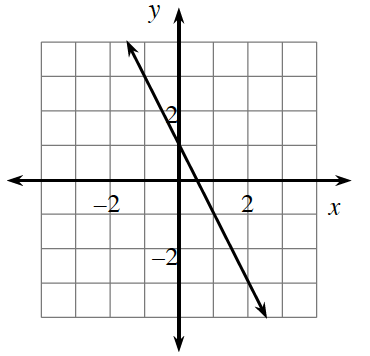### Home > CCAA8 > Chapter 2 Unit 2 > Lesson CCA: 2.2.2 > Problem2-65

2-65.

Use the $y$-intercept and the slope to write an equation of the line in the form of $y=mx+b$. (Create a slope triangle if you need one).
2. What are its $x$- and $y$-intercepts?
Remember that the $x$-intercept is the point on the graph where $y=0$ and that the $y$-intercept is the point where $x=0$.Use the eTool below to find the equation and determine the $x$ and $y$-intercepts.